## 0 前言

🔥 优质竞赛项目系列，今天要分享的是

🧿 更多资料, 项目分享：

## 1 实现方法

### 1.1 原理

##### 1.1.1 字符定位

gray_id_card_img = cv2.cvtColor(color_img, cv2.COLOR_BGR2GRAY)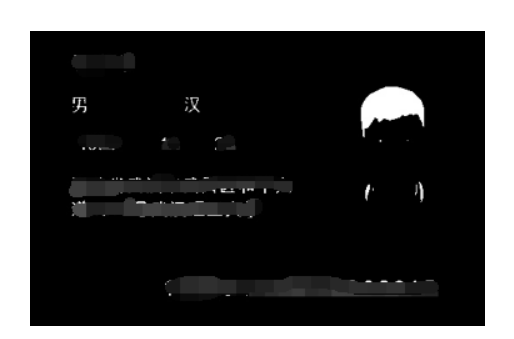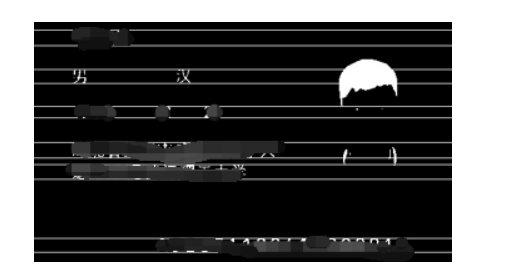`````` vertical_peek_ranges = extract_peek_ranges_from_array(
vertical_sum,
minimun_val=40,
minimun_range=1)
vertical_peek_ranges2d.append(vertical_peek_ranges)
``````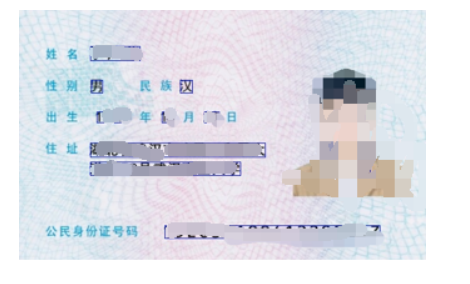##### 1.1.2 字符识别

1）身份证图像涉及个人隐私，很难获取其数据训练集。针对此问题，我采用获取身份证上印刷体汉字和数字的数据训练集的方法，利用Python图像库（PIL）将13类汉字印刷体字体转换成6492个类别，建立了较大的字符训练集；

2）如何获取身份证图片上的字符是在设计中一个重要问题。我采用水平和垂直投影技术，首先对身份证图像进行预处理，然后对图片在水平和垂直方向上像素求和，区分字符与空白区域，完成了身份证图像中字符定位与分割工作，有很好的切分效果；

3）在模型训练中模型的选择与设计是一个重要的环节，本文选择Lenet模型，发现模型层次太浅，然后增加卷积层和池化层，设计出了改进的深层Lenet模型，然后采用Caffe深度学习工具对模型进行训练，并在训练好的模型上进行测试，实验表明，模型的测试精度达到96.2%。

##### 1.1.3 深度学习算法介绍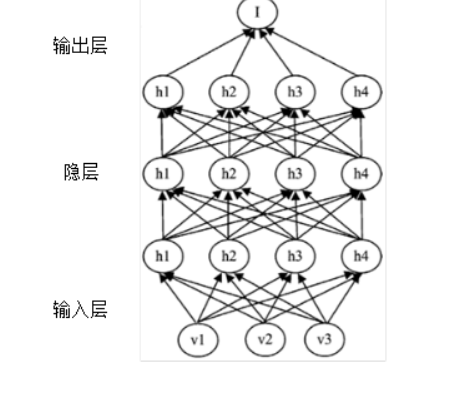##### 1.1.4 模型选择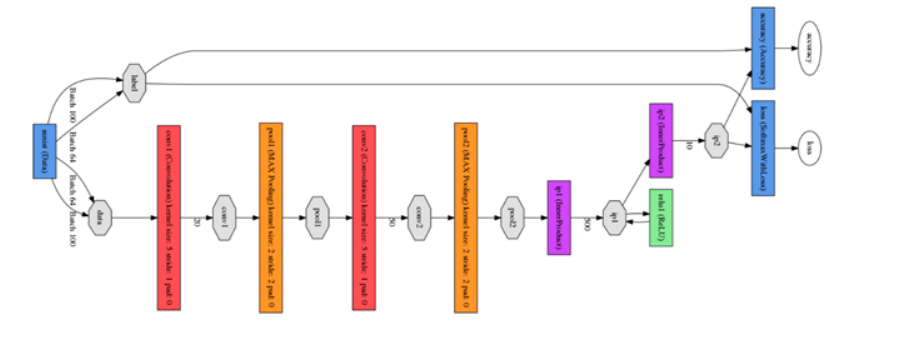### 2 算法流程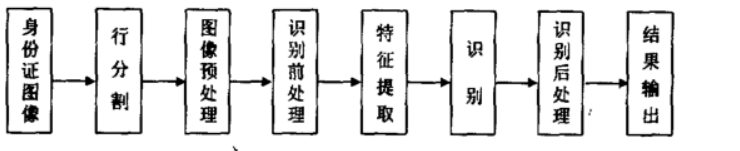### 3 部分关键代码

``````

##放大图片
resize_keep_ratio = PreprocessResizeKeepRatio(1024, 1024)
cv2_color_img = resize_keep_ratio.do(cv2_color_img)
##转换成灰度图
cv2_img = cv2.cvtColor(cv2_color_img, cv2.COLOR_RGB2GRAY)
height, width = cv2_img.shape
##二值化  调整自适应阈值 使得图像的像素值更单一、图像更简单
cv2_img, ##原始图像
255,     ##像素值上限
cv2.THRESH_BINARY,  ##赋值方法（二值化）
11,  ## 规定领域大小（一个正方形的领域）
2)   ## 常数C，阈值等于均值或者加权值减去这个常数

## 水平方向求和，找到行间隙和字符所在行（numpy）
## 根据求和结果获取字符行范围
peek_ranges = extract_peek_ranges_from_array(horizontal_sum)
vertical_peek_ranges2d = []
for peek_range in peek_ranges:
start_y = peek_range  ##起始位置
end_y = peek_range    ##结束位置
## 垂直方向求和，分割每一行的每个字符
vertical_sum = np.sum(line_img, axis=0)
## 根据求和结果获取字符行范围
vertical_peek_ranges = extract_peek_ranges_from_array(
vertical_sum,
minimun_val=40, ## 设最小和为40
minimun_range=1)  ## 字符最小范围为1
## 开始切割字符
vertical_peek_ranges = median_split_ranges(vertical_peek_ranges)
## 存放入数组中
vertical_peek_ranges2d.append(vertical_peek_ranges)

## 去除噪音，主要排除杂质，小的曝光点不是字符的部分
filtered_vertical_peek_ranges2d = []
for i, peek_range in enumerate(peek_ranges):
new_peek_range = []
median_w = compute_median_w_from_ranges(vertical_peek_ranges2d[i])
for vertical_range in vertical_peek_ranges2d[i]:
## 选取水平区域内的字符，当字符与字符间的间距大于0.7倍的median_w，说明是字符
if vertical_range - vertical_range > median_w*0.7:
new_peek_range.append(vertical_range)
filtered_vertical_peek_ranges2d.append(new_peek_range)
vertical_peek_ranges2d = filtered_vertical_peek_ranges2d

char_imgs = []
crop_zeros = PreprocessCropZeros()
resize_keep_ratio = PreprocessResizeKeepRatioFillBG(
norm_width, norm_height, fill_bg=False, margin=4)
for i, peek_range in enumerate(peek_ranges):
for vertical_range in vertical_peek_ranges2d[i]:
## 划定字符的上下左右边界区域
x = vertical_range
y = peek_range
w = vertical_range - x
h = peek_range - y
## 生成二值化图
## 输出二值化图
char_img = crop_zeros.do(char_img)
char_img = resize_keep_ratio.do(char_img)
## 加入字符图片列表中
char_imgs.append(char_img)
## 将列表转换为数组
np_char_imgs = np.asarray(char_imgs)

## 放入模型中识别并返回结果
output_tag_to_max_proba = caffe_cls.predict_cv2_imgs(np_char_imgs)

ocr_res = ""
## 读取结果并展示
for item in output_tag_to_max_proba:
ocr_res += item
print(ocr_res.encode("utf-8"))

## 生成一些Debug过程产生的图片
if debug_dir is not None:

#        color = (255, 0, 0)
#        for rect in rects:
#            x, y, w, h = rect
#            pt1 = (x, y)
#            pt2 = (x + w, y + h)

color = (0, 255, 0)
for i, peek_range in enumerate(peek_ranges):
for vertical_range in vertical_peek_ranges2d[i]:
x = vertical_range
y = peek_range
w = vertical_range - x
h = peek_range - y
pt1 = (x, y)
pt2 = (x + w, y + h)

debug_dir_chars = os.path.join(debug_dir, "chars")
os.makedirs(debug_dir_chars)
for i, char_img in enumerate(char_imgs):
path_char = os.path.join(debug_dir_chars, "%d.jpg" % i)
cv2.imwrite(path_char, char_img)

``````

## 4 效果展示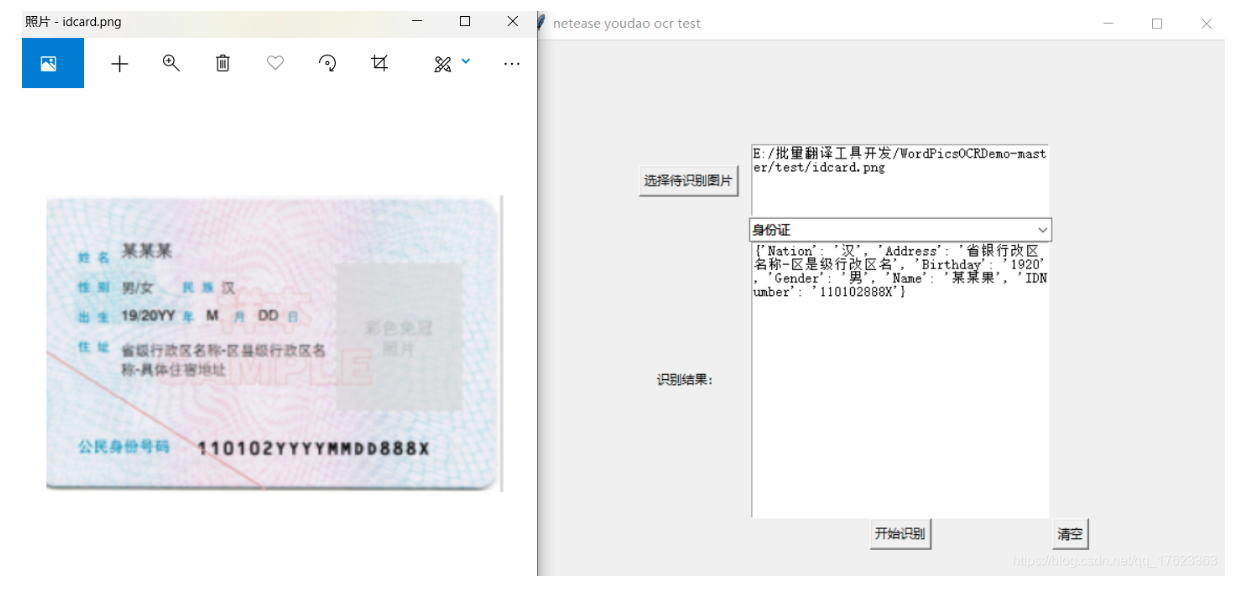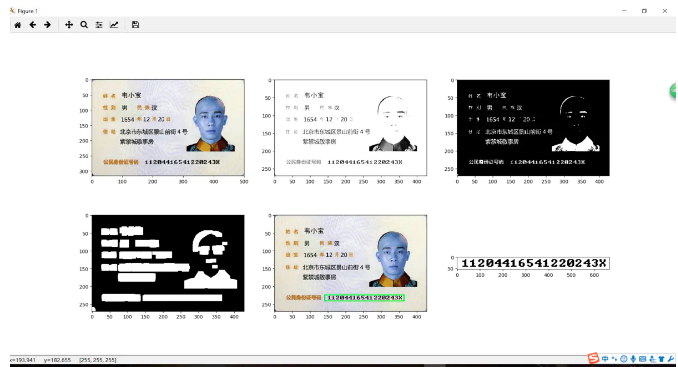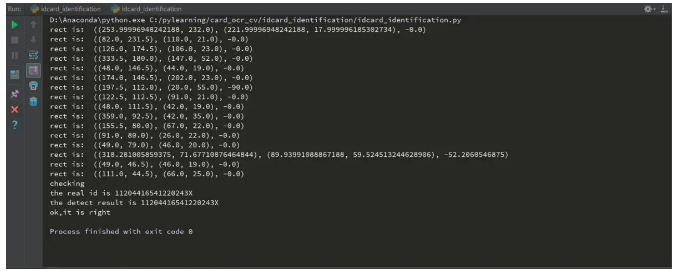## 5 最后

🧿 更多资料, 项目分享：